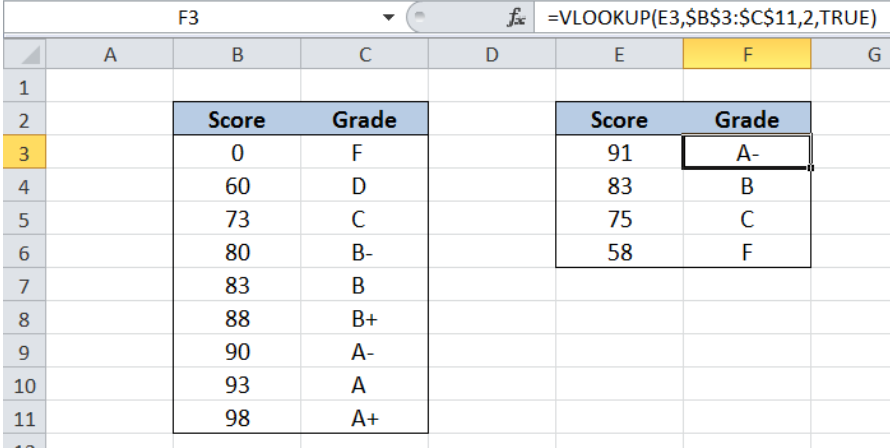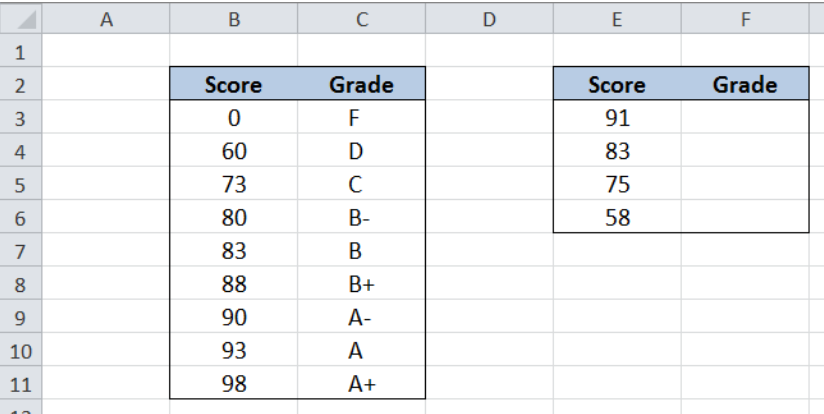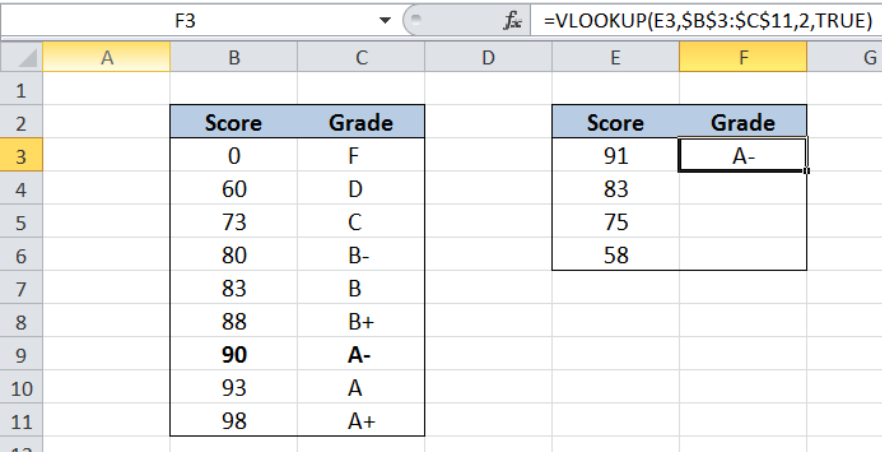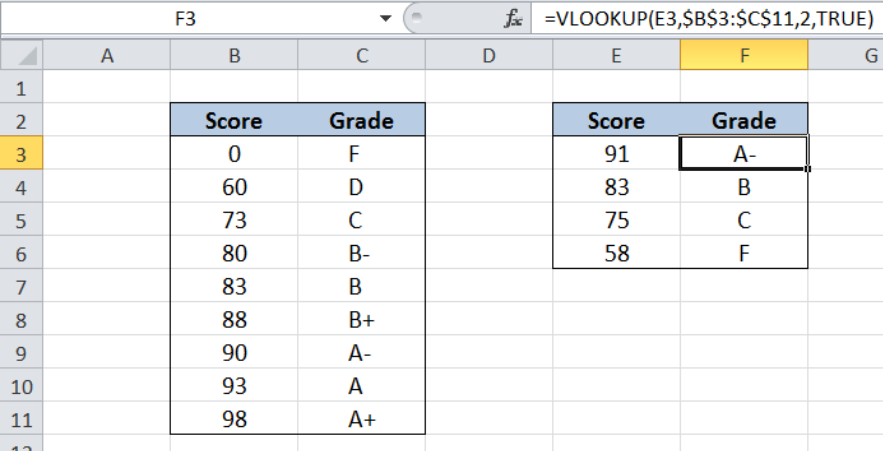Get instant live expert help with Excel or Google Sheets“My Excelchat expert helped me in less than 20 minutes, saving me what would have been 5 hours of work!”

#### Post your problem and you’ll get Expert help in seconds.

Your message must be at least 40 characters
Our professional Expert are available now. Your privacy is guaranteed.

# How to Calculate Grades with VLOOKUP in Excel

While working with Excel, we are able to lookup a value and retrieve data from a data set using the VLOOKUP function.  VLOOKUP provides a quick way of looking up a value from any list or range.  This step by step tutorial will assist all levels of Excel users to calculate grades with VLOOKUP.Figure 1. Final result: Calculate grades with VLOOKUP

Final formula:  `=VLOOKUP(E3,\$B\$3:\$C\$11,2,TRUE)`

## Syntax of the VLOOKUP function

`=VLOOKUP(lookup_value, table_array, col_index_num, [range_lookup])`

The parameters of the VLOOKUP function are:

• lookup_value – the value that we want to search and find in the table_array
• table_array – the range of cells in the source table containing the data we want to retrieve
• col_index_num the column number in the table_array corresponding to the information we want to retrieve, relative to the lookup_value
• [range_lookup] – optional; value is either TRUE or FALSE
• if TRUE or omitted, VLOOKUP returns either an exact or approximate match
• if FALSE, VLOOKUP will only find an exact match

## Setting up Our Data

Our data consists of two columns:  Score (column B) and Grade (column C).  In cells E3 to E6, we enter the scores we want to look up from our data in B3:C11.  In cells F3 to F6, we want to determine the corresponding grade for each given score by using VLOOKUP with an approximate match.Figure 2. Sample data  to calculate grades with VLOOKUP

It is important to remember that the table must be sorted in ascending order for the VLOOKUP formula to function properly with an approximate match.

We want to determine the corresponding grade for the given scores in column E.  In order to look up the scores and obtain the grade, we will be using VLOOKUP with an approximate match.  Let us follow these steps:

Step 1.  Select cell F3

Step 2.  Enter the formula: `=``VLOOKUP(E3,\$B\$3:\$C\$11,2,TRUE)`

Step 3:  Press ENTER

Step 4:  Copy the formula in cell F3 to cells F4:F6 by clicking the “+” icon at the bottom-right corner of cell F3 and dragging it down

The dollar signs “\$” in the formula fix the cells so that we can easily copy and paste the formula to other cells.Figure 3. Lookup grades using VLOOKUP and approximate match

Our lookup_value is cell E3, which contains the score we want to search for: “91”.  Our table_array is the range B3:C11. “Grade” is in the second column of the table array so col_index_num is 2.  Range_lookup is TRUE because we want to find either an exact or approximate match.

Our formula searches for the value 91 in the first column of our lookup table B3:C11, then returns the corresponding grade in column C.  If it doesn’t find an exact match, it will return the value closest to and less than the lookup value.

In our example, since 91 cannot be found in column B, our formula returns the grade for the value less than 91, which is 90.  As a result, the grade in cell F3 is A-.

Copying our formula to cells F4 to F6 returns the grades for the scores 86, 75 and 58.Figure 4. Output: Calculate grades with VLOOKUP

Most of the time, the problem you will need to solve will be more complex than a simple application of a formula or function. If you want to save hours of research and frustration, try our live Excelchat service! Our Excel Experts are available 24/7 to answer any Excel question you may have. We guarantee a connection within 30 seconds and a customized solution within 20 minutes.

### Did this post not answer your question? Get a solution from connecting with the expert.Another blog reader asked this question today on Excelchat:
Solution examplesI filtered data in column A (it is labeled ID #) of a data set. On my next worksheet, column A is also the ID #, but it is a different data set. I want to filter the ID #'s the same for the two sheets, but how do I do that being that I am working with two different sets of data?
Solved by V. H. in 22 minsI'm looking for a formula or format for if there is data in c4 on a sheet... It adds extra information underneath
Solved by X. E. in 20 minshi, my vlookup wont work in some rows but does in others. its temperemental. e.g it will work on rows 10 to 15, then not row 16 and 17, but the will for the rest.
Solved by K. Q. in 21 minsUse the Vlookup Function to complete the "employee" column of table 2. Use "job Id" from table 2 as your lookup_value(s) and table 1 as your reference.
Solved by C. H. in 16 minsIf a cell in another sheet is populated I need a vlookup done. If the cell is not populated I need the cell to return blank.
Solved by T. D. in 60 mins## Subscribe to Excelchat.coAnother blog reader asked this question today on Excelchat: